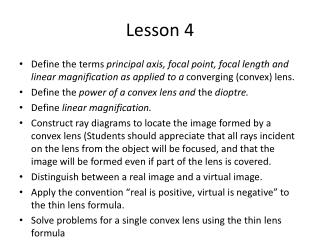# Lesson 4 - PowerPoint PPT PresentationDownload PresentationLesson 4

Lesson 4Download Presentation## Lesson 4

- - - - - - - - - - - - - - - - - - - - - - - - - - - E N D - - - - - - - - - - - - - - - - - - - - - - - - - - -
##### Presentation Transcript

1. Lesson 4 • Define the terms principal axis, focal point, focal length and linear magnification as applied to a converging (convex) lens. • Define the power of a convex lens and the dioptre. • Define linear magnification. • Construct ray diagrams to locate the image formed by a convex lens (Students should appreciate that all rays incident on the lens from the object will be focused, and that the image will be formed even if part of the lens is covered. • Distinguish between a real image and a virtual image. • Apply the convention “real is positive, virtual is negative” to the thin lens formula. • Solve problems for a single convex lens using the thin lens formula

2. Lenses

3. Converging and diverging lenses

4. Principal axis The straight line that goes through the centre of the lens at right angles to the lens surface

5. Focal point Rays parallel to the principal axis, after being refracted by the lens, will all pass through a point on the principal axis called the focal point.

6. Focal length The distance from the focal point to the centre of the lens (denoted by the symbol f)

7. Finding the focal length - a quick practical

8. Power of a lens The power of a lens is defined as the inverse focal length. Power is measured in dioptres. 1 D = 1 m-1.

9. Ray diagrams

10. Standard ray 1 A ray parallel to the principal axis will go through the focal point.

11. Standard ray 2 A ray passing through the left focal point will emerge parallel to the principal axis

12. Standard ray 3 A ray passing through the centre of the lens will emerge undeflected

13. Ray diagrams With the help of these 3 standard rays we can find the image of any object placed in front of a convex lens

14. Real and virtual images • A real image is where the rays actually pass through the image and it can be projected and seen on a screen

15. Real and virtual images • A virtual image is where no rays of light pass through, only their mathematical extensions. It cannot be displayed on a screen

16. Let’s try it! – Investigating images practical

17. Why? Let’s construct some ray diagrams to find out!

18. Thin lens formula

19. Thin lens formula • f is positive for a converging lens • u is positive • v is positive for real images and negative for virtual images • M > 0 means the image is upright • M < 0 means the image is inverted

20. Linear magnification Linear magnification is defined as the ratio of the image height to the object height

21. Example problem • A converging lens has a focal length of 15cm. An object is placed 60 cm from the lens. Determine the image.

22. Example problem • A converging lens has a focal length of 15cm. An object is placed 60 cm from the lens. Determine the image. • f = 0.15, u = 0.6 • 1/f = 1/u + 1/v • 1/v = 1/f – 1/u = 1/0.15 – 1/0.6 = 1/0.2 • v = 0.2 m • The image is real (positive v), m = -0.2/0.6 = -1/3 which means the image is inverted and smaller than the object.

23. Questions • Page 621 questions 6, 8, 9, 10, 12.